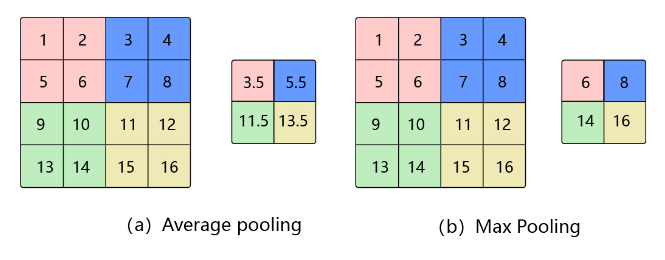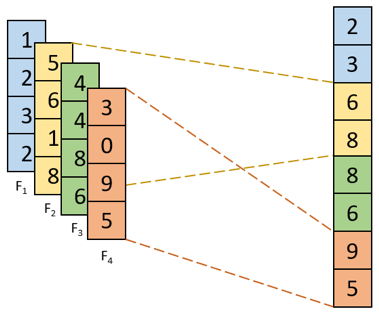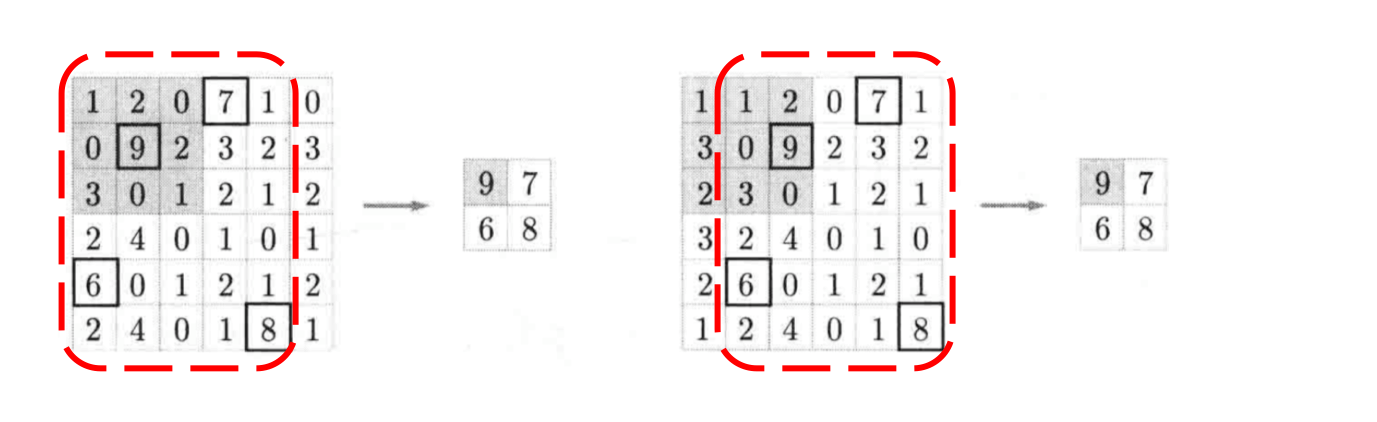# 池化¶

## 1 基础概念¶• 平均池化： 计算区域子块所包含所有像素点的均值，将均值作为平均池化结果。如 图1(a)，这里使用大小为$$2\times2$$的池化窗口，每次移动的步幅为2，对池化窗口覆盖区域内的像素取平均值，得到相应的输出特征图的像素值。池化窗口的大小也称为池化大小，用$$k_h \times k_w$$表示。在卷积神经网络中用的比较多的是窗口大小为$$2 \times 2$$，步幅为2的池化。

• 最大池化： 从输入特征图的某个区域子块中选择值最大的像素点作为最大池化结果。如 图1(b)，对池化窗口覆盖区域内的像素取最大值，得到输出特征图的像素值。当池化窗口在图片上滑动时，会得到整张输出特征图。• K-max池化： 对输入特征图的区域子块中像素点取前K个最大值，常用于自然语言处理中的文本特征提取。如图2，从包含了4个取值的每一列中选取前2个最大值就得到了K最大池化结果。

## 2 特点¶

1. 当输入数据做出少量平移时，经过池化后的大多数输出还能保持不变，因此，池化对微小的位置变化具有鲁棒性。例如 图3 中，输入矩阵向右平移一个像素值，使用最大池化后，结果与平移前依旧能保持不变。图3 微小位置变化时的最大池化结果

2. 由于池化之后特征图会变小，如果后面连接的是全连接层，能有效的减小神经元的个数，节省存储空间并提高计算效率

## 3 池化中填充的方式¶

### 3.1 int输入¶

int输入即接收一个int类型的数字n，对图片的四周包裹n行n列的0来填充图片。如果要保持图片尺寸不变，n的值和池化窗口的大小是有关的。假如 $$H_{in}, W_{in}$$ 为图片输入的大小，$$k_h, k_w$$ 为池化窗口的大小，$$H_{out}, H_{out}$$ 为结果图的大小的话，他们之间有着这样的关系。 $$$H_{out} = \frac{H_{in} + 2*p_h - k_h}{s_h} + 1 \\ W_{out} = \frac{W_{out} + 2*p_w -k_w}{s_w} + 1$$$$在使用3×3的池化窗口且步长为1的情况下，还要保持图片大小不变，则需要使用padding=1的填充。 那么，公式就变为了$$$$H_{out} = \frac{6 - 3 + 2*1}{1} + 1 \\ W_{out} = \frac{6 - 3 + 2*1}{1} + 1$$$$另外，在stride不为1且不能被整除的情况下，整体结果向下取整。 关于Padding和K的公式如下$$$$Padding = \frac{(k-1)}{2} \quad \quad (k \% 2 != 0)$$$

import paddle # No padding
x = paddle.rand((1, 1, 6, 6))
y = avgpool(x)
print('result:', 'shape of x:', x.shape, 'shape of result:', y.shape)

result: shape of x: [1, 1, 6, 6] shape of result: [1, 1, 4, 4]


import paddle # Padding 1
x = paddle.rand((1, 1, 6, 6))
y = avgpool(x)
print('result:', 'shape of x:', x.shape, 'shape of result:', y.shape)

result: shape of x: [1, 1, 6, 6] shape of result: [1, 1, 6, 6]


### 3.2 list和tuple输入¶

import paddle # No padding and different kernel size
x = paddle.rand((1, 1, 12, 6)) # 12为高H， 6为宽W
y = avgpool(x)
print('result:', 'shape of x:', x.shape, 'shape of result:', y.shape)
result: shape of x: [1, 1, 12, 6] shape of result: [1, 1, 10, 2]


import paddle # No padding and different kernel size
x = paddle.rand((1, 1, 12, 6)) # 12为高H， 6为宽W
y = avgpool(x)
print('result:', 'shape of x:', x.shape, 'shape of result:', y.shape)
result: shape of x: [1, 1, 12, 6] shape of result: [1, 1, 12, 6]


### 3.3 string输入¶

string输入有两个值，一个是SAME，一个是VALID。这两个的计算公式如下：

SAME:$$H_{out} = \lceil \frac{H_{in}}{s_h} \rceil$$, $$W_{out} = \lceil\frac{W_{in}}{s_w}\rceil$$

VALID:$$H_{out} = \frac{H_{in} - k_h}{s_h} + 1$$, $$W_{out} = \frac{W_{in} - k_w}{s_w} + 1$$

import paddle # Padding SAME kernel_size 2
x = paddle.rand((1, 1, 6, 6))
y = avgpool(x)
print('result:', 'shape of x:', x.shape, 'shape of result:', y.shape)
result: shape of x: [1, 1, 6, 6] shape of result: [1, 1, 3, 3]


import paddle # Padding SAME kernel_size 1
x = paddle.rand((1, 1, 6, 6))
y = avgpool(x)
print('result:', 'shape of x:', x.shape, 'shape of result:', y.shape)
result: shape of x: [1, 1, 6, 6] shape of result: [1, 1, 6, 6]


import paddle # Padding VALID
x = paddle.rand((1, 1, 6, 6))
y = avgpool(x)
print('result:', 'shape of x:', x.shape, 'shape of result:', y.shape)
result: shape of x: [1, 1, 6, 6] shape of result: [1, 1, 3, 3]


## 4 应用示例¶

$H_{out} = \frac{H + p_{h1} + p_{h2} - k_h}{s_h} + 1$
$W_{out} = \frac{W + p_{w1} + p_{w2} - k_w}{s_w} + 1$

$H_{out} = \frac{H}{2}$
$W_{out} = \frac{W}{2}$

$H_{out} = \frac{H + p_{h1} + p_{h2} - k_h}{s_h} + 1=\frac{4 + 0 + 0 - 2}{2} + 1=\frac{4}{2}=2$
$W_{out} = \frac{W + p_{w1} + p_{w2} - k_w}{s_w} + 1=\frac{4 + 0 + 0 - 2}{2} + 1=\frac{4}{2}=2$

1. 池化窗口的初始位置为左上角，对应粉色区域，此时输出为 $$3.5 = \frac{1 + 2 + 5 + 6}{4}$$

2. 由于步幅为2，所以池化窗口向右移动两个像素，对应蓝色区域，此时输出为 $$5.5 = \frac{3 + 4 + 7 + 8}{4}$$

3. 遍历完第一行后，再从第三行开始遍历，对应绿色区域，此时输出为 $$11.5 = \frac{9 + 10 + 13 + 14}{4}$$

4. 池化窗口向右移动两个像素，对应黄色区域，此时输出为 $$13.5 = \frac{11 + 12 + 15 + 16}{4}$$